Name:    Quiz 2.3-2.4

Multiple Choice
Identify the choice that best completes the statement or answers the question.

Subtract.  Write fractions in simplest form.

1.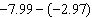a. –5 c. –5.02 b. –4.52 d. 5.02

2.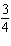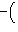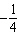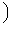a.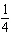c.b.d.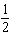3.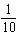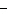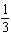a. –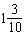c.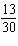b.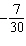d.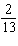4.

–4.7 – 0.3
 a. –4.4 c. –5 b. 4.4 d. 5

5.

Find the distance between the two numbers on a number line.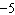,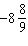a.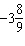c.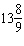b.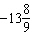d.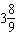6.

Find the distance between the two numbers on a number line.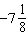,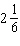a.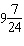c.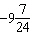b.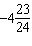d.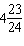Divide. Write your answer in simplest form.

7.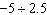a. –0.5 c. –2 b. 2 d. 7.5

8.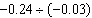a. 8 c. 1.25 b. –0.125 d. 80

9.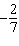¸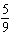a.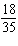c.b.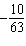d.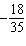10.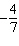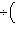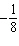a.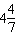c.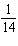b.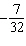d.Multiply.  Write your fractions in simplest form.

11.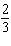´a.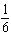c. –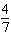b.d. –12.

–7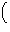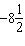a.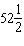c.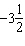b.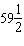d. –1

13.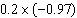a. –0.194 c. –0.213 b. –0.206 d. 0.194

14.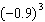a. –2.7 c. 0.729 b. –0.729 d. –72.9

Numeric Response

1.

The table shows four bank account transactions. Find the change in the account balance.

 Transactions Deposit \$85.00 Withdrawal –\$40.00 Game Rentals –\$65.00 Deposit \$65.00

2.

How many 0.5-pound packages can be made with 7 pounds of sunflower seeds?

3.

A 17.2 gallon gasoline tank isfull. How many gallons will it take to fill the tank?

A cook has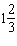cups of flour. A recipe calls for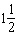cups of flour. Does the cook have enough flour? If not, how much more flour is needed?